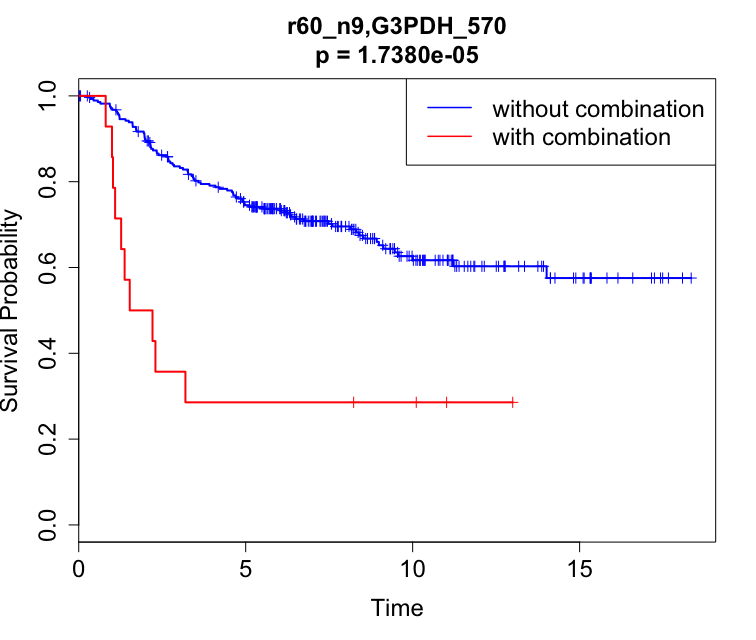# LAMP for Survival Analysis

View the Project on GitHub rtrelator/SurvivalLAMP

Survival LAMP is an extended version of LAMP (Terada et al 2013) for performing multiple testing correction in finding combinatorial markers using log-rank test in survival analysis. Details and usage of the original LAMP can be found here.

### How to run LAMP for survival analysis:

2. Compile: run `make` in corresponding directory
3. Run:
``````python lampSA.py -p logrank [item_file] [status_file] [significance_level] -t [time_file] > [output_file]
``````

EXAMPLE:

``````python lampSA.py -p logrank sample/sample_logrank_item.csv sample/sample_logrank_status.csv 0.05 -t sample/sample_logrank_time.csv > sample/sample_logrank_output.txt
``````

### Input Files:

1. ITEM FILE
• similar format to the original LAMP item file, this file contains association information of samples/individuals and markers
• first row as header (commented first entry, contains marker identifiers)
• first column contains the list of sample/individual IDs (one sample/individual per row)
• succeeding columns represent markers (e.g. gene, SNPs, etc.) (one marker per column)
• entries are 1 or 0 (integer type)
• file format: csv
• example: sample/sample_logrank_item.csv
2. STATUS FILE
• similar format to the original LAMP value file, except that it contains the status of samples/individuals
• first row as header (commented first entry similar to item file)
• first column must be the same as the item file
• second column contains status of corresponding samples/individuals (1 = event, 0 = censored)
• file format: csv
• example: sample/sample_logrank_status.csv
3. TIME FILE
• contains survival time information of samples/individuals
• first row as header (commented first entry similar to item and status files)
• first column must be the same as the item and value files
• second column contains survival time of corresponding samples/individuals (e.g. in months, years, or days)
• file format: csv
• example: sample/sample_logrank_time.csv

### Output File:

• similar format to the original LAMP output file
• example: sample/sample_logrank_output.txt
``````# Survival LAMP ver. 1.0
# item-file: sample/sample_logrank_item.csv
# value-file: sample/sample_logrank_status.csv
# time-file: sample/sample_logrank_time.csv
# significance-level: 0.05
# P-value computing procedure: logrank
# # of tested elements: 9, # of samples: 292, # of positive samples: 101
# Adjusted significance level: 0.0005618, Correction factor: 89 (# of target rows >= 1)
# # of significant combinations: 5
Rank	Raw p-value	Adjusted p-value	Combination	Arity	# of target rows	# of failed targets
1	1.738e-05	0.0015468	r60_n9,G3PDH_570	2	14	10
2	2.4931e-05	0.0022188	r60_n9,G3PDH_570,r60_1,r60_3	4	9	7
3	8.6793e-05	0.0077246	r60_n9,G3PDH_570,r60_3	3	11	8
4	0.00039433	0.035096	r60_n9,r60_a22,G3PDH_570	3	10	7
5	0.00042816	0.038106	r60_n9,Pro25G,G3PDH_570	3	10	7
Time (sec.): Computing correction factor 0.620, P-value 1.363, Total 1.983
``````
• # of tested elements = number of marker columns in item file
• # of samples = number of samples/individuals included (not counting censored samples/individuals after first failure time)
• # of positive samples = number of failed samples/individuals (i.e. status = 1)
• # of target rows = number of samples/individuals affected by the combination
• # of failed targets = number of samples/individuals affected by the combination whose status is 1 (i.e. failures)

### Log-rank Test and Kaplan-Meier Curves in R

Performing log-rank test and generating KM plots for the combination results can be implemented using the survival package in R:

#### Log-rank Test

``````library(survival)

# read the three input files for LAMP
df = read.table("sample/sample_logrank_item.csv", sep = ",", header = T, comment.char = "", check.names = F, stringsAsFactors = F)
df_status = read.table("sample/sample_logrank_status.csv", sep = ",", header = T, comment.char = "", check.names = F, stringsAsFactors = F)
df_time = read.table("sample/sample_logrank_time.csv", sep = ",", header = T, comment.char = "", check.names = F, stringsAsFactors = F)

# can join into one data frame, otherwise use respective columns of each data frame separately
df\$TIME = df_time\$TIMErecurrence; df\$STATUS = df_status\$EVENTrecurrence

# compute for combination value by getting the product for each sample/individual, add to data frame as column 'COMB'
# example for combination r60_n9,G3PDH_570
df\$COMB = apply(df[,c("r60_n9","G3PDH_570")], 1, prod)

# perform log-rank test
lr = survdiff(Surv(TIME, STATUS) ~ COMB, data = df)
print(lr)

Call:
survdiff(formula = Surv(TIME, STATUS) ~ COMB, data = df)

N Observed Expected (O-E)^2/E (O-E)^2/V
COMB=0 281       91    98.14      0.52      18.5
COMB=1  14       10     2.86     17.87      18.5

Chisq= 18.5  on 1 degrees of freedom, p= 1.74e-05
``````

In the R results:

• COMB=0: population not containing the corresponding marker combination (i.e. item file value = 0 for at least one of the markers)
• COMB=1: population containing the corresponding marker combination (i.e. item file value = 1 for all of the markers)
• N >= # of target rows in the LAMP results (since LAMP disregards censored samples before first failure time. However, this has no effect on the resulting p-value)
• Observed = # of failed targets in the LAMP results
• p = raw log-rank p-value of the combination

#### Plotting KM Curves

``````# fit Kaplan-Meier and plot curves
fit = survfit(Surv(TIME, STATUS) ~ COMB, data = df)
plot(fit)

# add tick marks for censored data and color legends
plot(fit, mark.time = T, col = c("blue", "red"), xlab = "Time", ylab = "Survival Probability", lwd = 2, lty = 1, cex.lab = 1.5, cex.axis = 1.5, cex.main = 1.5, cex.sub = 1.5)
legend("topright", c("without combination", "with combination"), lty = 1, col = c("blue","red"), lwd = 1.5, cex = 1.5)

# getting p-value from log-rank test result
pval = 1 - pchisq(lr\$chisq, 1)
title(sprintf("r60_n9,G3PDH_570\np = %.4e", pval), cex.main = 1.5)
``````

The code above will produce the following plot:### Reference

Raissa T. Relator, Aika Terada, Jun Sese. Identifying statistically significant combinatorial markers for survival analysis. BMC Medical Genomics. 2018 11(Suppl 2):31.